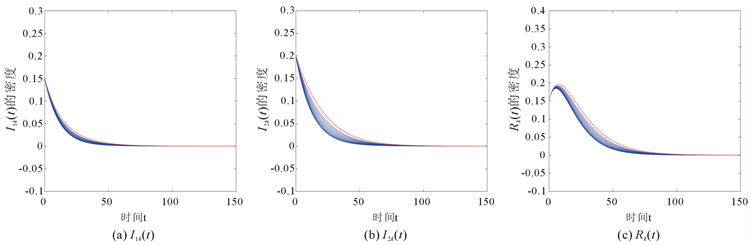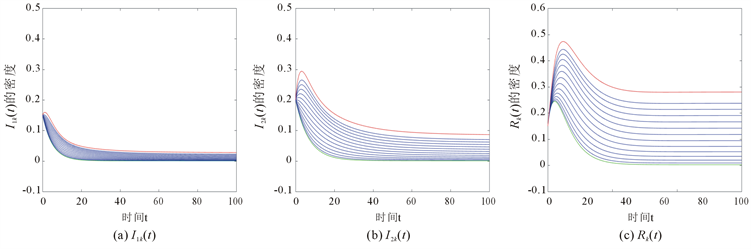#### 期刊菜单

Dynamics Analysis of an SIRS Epidemic Model on Weighted Networks
DOI: 10.12677/AAM.2019.83042, PDF, HTML, XML, 下载: 906  浏览: 1,518  科研立项经费支持

Abstract: In this paper, an improved SIRS epidemic model on weighted networks is investigated and we ob-tain that the basic reproduction number R0 determines whether a disease persists, i.e., if R0<1, then the disease-free equilibrium E0 is globally asymptotically stable; if R0>1, there exists a unique endemic equilibrium E*. Furthermore, the model is quasi-persistent if R0>1. Finally, some simulations are presented to demonstrate the correctness of the theoretical results.

1. 引言

2. 传染病模型

${S}_{k}\left(t\right),{I}_{1k}\left(t\right),{I}_{2k}\left(t\right),{R}_{k}\left(t\right)$ 分别表示为t时刻度为k的易感个体的密度，低风险染病个体的密度，

$\left(\begin{array}{l}\frac{\text{d}{S}_{k}\left(t\right)}{\text{d}t}=-k{S}_{k}\left(t\right)\Theta \left(t\right)+\delta {R}_{k}\left(t\right),\\ \frac{\text{d}{I}_{1k}\left(t\right)}{\text{d}t}=\alpha k{S}_{k}\left(t\right)\Theta \left(t\right)-{\gamma }_{1}{I}_{1k}\left(t\right),\\ \frac{\text{d}{I}_{2k}\left(t\right)}{\text{d}t}=\left(1-\alpha \right)k{S}_{k}\left(t\right)\Theta \left(t\right)-{\gamma }_{2}{I}_{2k}\left(t\right),\\ \frac{\text{d}{R}_{k}\left(t\right)}{\text{d}t}={\gamma }_{1}{I}_{1k}\left(t\right)+{\gamma }_{2}{I}_{2k}\left(t\right)-\delta {R}_{k}\left(t\right),\end{array}$ (1)

$\begin{array}{l}{\Theta }_{1}\left(t\right)=\underset{i=1}{\overset{n}{\sum }}P\left(i|k\right)\frac{{\phi }_{1}\left(i\right)}{i}{\lambda }_{ik}{I}_{1i}\left(t\right)=\frac{1}{〈k〉}\underset{i=1}{\overset{n}{\sum }}\text{ }{\lambda }_{ik}{\phi }_{1}\left(i\right)P\left(i\right){I}_{1i}\left(t\right),\\ {\Theta }_{2}\left(t\right)=\underset{i=1}{\overset{n}{\sum }}P\left(i|k\right)\frac{{\phi }_{2}\left(i\right)}{i}{\lambda }_{ik}{I}_{1i}\left(t\right)=\frac{1}{〈k〉}\underset{i=1}{\overset{n}{\sum }}\text{ }{\lambda }_{ik}{\phi }_{2}\left(i\right)P\left(i\right){I}_{2i}\left(t\right),\end{array}$ (2)

${\lambda }_{ik}=\lambda i\frac{\varphi \left(i,k\right)}{{\Psi }_{i}}=\frac{\lambda g\left(k\right)〈k〉}{〈kg\left(k\right)〉}.$ (3)

${\stackrel{¯}{\Theta }}_{1}\left(t\right)=\frac{\lambda g\left(k\right)}{〈kg\left(k\right)〉}\underset{i=1}{\overset{n}{\sum }}\text{ }{\phi }_{1}\left(i\right)P\left(i\right){I}_{1i}\left(t\right),{\stackrel{¯}{\Theta }}_{2}\left(t\right)=\frac{\lambda g\left(k\right)}{〈kg\left(k\right)〉}\underset{i=1}{\overset{n}{\sum }}\text{ }{\phi }_{2}\left(i\right)P\left(i\right){I}_{2i}\left(t\right).$ (4)

$\left(\begin{array}{l}\frac{\text{d}{S}_{k}\left(t\right)}{\text{d}t}=-\frac{\lambda k{S}_{k}\left(t\right)g\left(k\right)}{〈kg\left(k\right)〉}\theta \left(t\right)+\delta {R}_{k}\left(t\right),\hfill \\ \frac{\text{d}{I}_{1k}\left(t\right)}{\text{d}t}=\frac{\alpha \lambda k{S}_{k}\left(t\right)g\left(k\right)}{〈kg\left(k\right)〉}\theta \left(t\right)-{\gamma }_{1}{I}_{1k}\left(t\right),\hfill \\ \frac{\text{d}{I}_{2k}\left(t\right)}{\text{d}t}=\frac{\left(1-\alpha \right)\lambda k{S}_{k}\left(t\right)g\left(k\right)}{〈kg\left(k\right)〉}\theta \left(t\right)-{\gamma }_{2}{I}_{2k}\left(t\right),\hfill \\ \frac{\text{d}{R}_{k}\left(t\right)}{\text{d}t}={\gamma }_{1}{I}_{1k}\left(t\right)+{\gamma }_{2}{I}_{2k}\left(t\right)-\delta {R}_{k}\left(t\right),\hfill \end{array}$ (5)

$\begin{array}{l}0\le {S}_{k}\left(0\right),{I}_{1k}\left(0\right),{I}_{2k}\left(0\right),{R}_{k}\left(0\right)\le 1,\\ {S}_{k}\left(0\right)+{I}_{1k}\left(0\right)+{I}_{2k}\left(0\right)+{R}_{k}\left(0\right)=1,\theta \left(0\right)>0,k=1,2,\cdots ,n.\end{array}$

$\left(\begin{array}{l}\frac{\text{d}{I}_{1k}\left(t\right)}{\text{d}t}=\frac{\alpha \lambda k\left(1-{I}_{1k}\left(t\right)-{I}_{2k}\left(t\right)-{R}_{k}\left(t\right)\right)g\left(k\right)}{〈kg\left(k\right)〉}\theta \left(t\right)-{\gamma }_{1}{I}_{1k}\left(t\right),\\ \frac{\text{d}{I}_{2k}\left(t\right)}{\text{d}t}=\frac{\left(1-\alpha \right)\lambda k\left(1-{I}_{1k}\left(t\right)-{I}_{2k}\left(t\right)-{R}_{k}\left(t\right)\right)g\left(k\right)}{〈kg\left(k\right)〉}\theta \left(t\right)-{\gamma }_{2}{I}_{2k}\left(t\right),\\ \frac{\text{d}{R}_{k}\left(t\right)}{\text{d}t}={\gamma }_{1}{I}_{1k}\left(t\right)+{\gamma }_{2}{I}_{2k}\left(t\right)-\delta {R}_{k}\left(t\right).\end{array}$ (6)

3. 动力学分析

${\mathcal{R}}_{0}=\frac{\alpha \lambda 〈k{\phi }_{1}\left(k\right)g\left(k\right)〉}{{\gamma }_{1}〈kg\left(k\right)〉}+\frac{\left(1-\alpha \right)\lambda 〈k{\phi }_{2}\left(k\right)g\left(k\right)〉}{{\gamma }_{2}〈kg\left(k\right)〉}.$

(i) 系统(6)总存在一个无病平衡点 ${E}_{0}=\left(\underset{3n个}{\underset{︸}{0,0,0,\cdots ,0,0,0}}\right)$

(ii) 当 ${\mathcal{R}}_{0}>1$ 时，系统(6)有且仅有唯一的正平衡点 ${E}^{*}=\left({I}_{11}^{*},{I}_{21}^{*},{R}_{1}^{*},\cdots ,{I}_{1n}^{*},{I}_{2n}^{*},{R}_{n}^{*}\right)$ 。并且 ${E}^{*}=\left({I}_{11}^{*},{I}_{21}^{*},{R}_{1}^{*},\cdots ,{I}_{1n}^{*},{I}_{2n}^{*},{R}_{n}^{*}\right)$ 满足

${I}_{1k}^{*}=\frac{\alpha \delta {\gamma }_{2}\lambda kg\left(k\right){\theta }^{*}}{{D}_{k}},\text{ }{I}_{2k}^{*}=\frac{\left(1-\alpha \right)\delta {\gamma }_{1}\lambda kg\left(k\right){\theta }^{*}}{{D}_{k}},\text{ }{R}_{k}^{*}=\frac{{\gamma }_{1}{\gamma }_{2}\lambda kg\left(k\right){\theta }^{*}}{{D}_{k}},$

$\left(\begin{array}{l}\frac{\alpha \lambda k\left(1-{I}_{1k}^{*}-{I}_{2k}^{*}-{R}_{k}^{*}\right)g\left(k\right)}{〈kg\left(k\right)〉}{\theta }^{*}-{\gamma }_{1}{I}_{1k}^{*}=0,\\ \frac{\left(1-\alpha \right)\lambda k\left(1-{I}_{1k}^{*}-{I}_{2k}^{*}-{R}_{k}^{*}\right)g\left(k\right)}{〈kg\left(k\right)〉}{\theta }^{*}-{\gamma }_{2}{I}_{2k}^{*}=0,\\ {\gamma }_{1}{I}_{1k}^{*}+{\gamma }_{2}{I}_{2k}^{*}-\delta {R}_{k}^{*}=0,\end{array}$ (7)

${I}_{2k}^{*}=\frac{{\gamma }_{1}\left(1-\alpha \right)}{{\gamma }_{2}\alpha }{I}_{1k}^{*}.$ (8)

${R}_{k}^{*}=\frac{{\gamma }_{1}}{\mu +\delta }{I}_{1k}^{*}+\frac{{\gamma }_{2}}{\mu +\delta }{I}_{2k}^{*}=\frac{{\gamma }_{1}}{\alpha \delta }{I}_{1k}^{*}.$ (9)

${I}_{1k}^{*}=\frac{\alpha \delta {\gamma }_{2}\lambda kg\left(k\right){\theta }^{*}}{{D}_{k}}.$ (10)

${\theta }^{*}=\underset{k=1}{\overset{n}{\sum }}\text{ }{\phi }_{1}\left(k\right)P\left(k\right)\frac{\alpha \delta {\gamma }_{2}\lambda kg\left(k\right){\theta }^{*}}{{D}_{k}}+\underset{k=1}{\overset{n}{\sum }}\text{ }{\phi }_{2}\left(k\right)P\left(k\right)\frac{\left(1-\alpha \right)\delta {\gamma }_{1}\lambda kg\left(k\right){\theta }^{*}}{{D}_{k}}.$ (11)

$f\left({\theta }^{*}\right)={\sum }_{k=1}^{n}\text{ }{\phi }_{1}\left(k\right)P\left(k\right)\frac{\alpha \delta {\gamma }_{2}\lambda kg\left(k\right)}{{D}_{k}}+{\sum }_{k=1}^{n}\text{ }{\phi }_{2}\left(k\right)P\left(k\right)\frac{\left(1-\alpha \right)\delta {\gamma }_{1}\lambda kg\left(k\right)}{{D}_{k}}$ ，然后方程(11)可写成如下

${\theta }^{*}={\theta }^{*}f\left({\theta }^{*}\right).$ (12)

$\left(\begin{array}{l}f\left(\theta \right)<1,\\ \frac{\text{d}f\left({\theta }^{*}\right)}{\text{d}{\theta }^{*}}=-\underset{k=1}{\overset{n}{\sum }}\text{ }{\phi }_{1}\left(k\right)P\left(k\right)\frac{\alpha \delta {\gamma }_{2}\lambda kg\left(k\right){A}_{k}}{{D}_{k}^{2}}-\underset{k=1}{\overset{n}{\sum }}\text{ }{\phi }_{2}\left(k\right)P\left(k\right)\frac{\left(1-\alpha \right)\delta {\gamma }_{1}\lambda kg\left(k\right){A}_{k}}{{D}_{k}^{2}}<0.\end{array}$

$\begin{array}{c}y\left(t\right)={\left({y}_{1}\left(t\right),{y}_{2}\left(t\right),\cdots ,{y}_{n}\left(t\right),{y}_{n+1}\left(t\right),{y}_{n+2}\left(t\right),\cdots ,{y}_{2n},{y}_{2n+1}\left(t\right),{y}_{2n+2}\left(t\right),\cdots ,{y}_{3n}\right)}^{\text{T}}\\ ={\left({I}_{11}\left(t\right),{I}_{12}\left(t\right),\cdots ,{I}_{1n}\left(t\right),{I}_{21}\left(t\right),{I}_{22}\left(t\right),\cdots ,{I}_{2n}\left(t\right),{R}_{1}\left(t\right),{R}_{2}\left(t\right),\cdots ,{R}_{n}\left(t\right)\right)}^{\text{T}},\end{array}$

$\frac{\text{d}y}{\text{d}t}=Ay+N\left(y\right),$ (13)

$A=\left(\begin{array}{ccc}{A}_{11}& {A}_{12}& O\\ {A}_{21}& {A}_{22}& O\\ {A}_{31}& {A}_{32}& {A}_{33}\end{array}\right),$

${A}_{11}=\left(\begin{array}{cccc}\alpha g\left(1\right){X}_{1}-{\gamma }_{1}& \alpha g\left(1\right){X}_{2}& \cdots & \alpha g\left(1\right){X}_{n}\\ 2\alpha g\left(2\right){X}_{1}& 2\alpha g\left(2\right){X}_{2}-{\gamma }_{1}& \cdots & 2\alpha g\left(2\right){X}_{n}\\ ⋮& ⋮& \ddots & ⋮\\ n\alpha g\left(n\right){X}_{1}& n\alpha g\left(n\right){X}_{2}& \cdots & n\alpha g\left(n\right){X}_{n}-{\gamma }_{1}\end{array}\right),$

${A}_{12}=\left(\begin{array}{cccc}\alpha g\left(1\right){Y}_{1}& \alpha g\left(1\right){Y}_{2}& \cdots & \alpha g\left(1\right){Y}_{n}\\ 2\alpha g\left(2\right){Y}_{1}& 2\alpha g\left(2\right){Y}_{2}& \cdots & 2\alpha g\left(2\right){Y}_{n}\\ ⋮& ⋮& \ddots & ⋮\\ n\alpha g\left(n\right){Y}_{1}& n\alpha g\left(\left(n\right){Y}_{2}& \cdots & n\alpha g\left(n\right){Y}_{n}\end{array}\right),$

${A}_{21}=\left(\begin{array}{cccc}\left(1-\alpha \right)g\left(1\right){X}_{1}& \left(1-\alpha \right)g\left(1\right){X}_{2}& \cdots & \left(1-\alpha \right)g\left(1\right){X}_{n}\\ 2\left(1-\alpha \right)g\left(2\right){X}_{1}& 2\left(1-\alpha \right)g\left(2\right){X}_{2}& \cdots & 2\left(1-\alpha \right)g\left(2\right){X}_{n}\\ ⋮& ⋮& \ddots & ⋮\\ n\left(1-\alpha \right)g\left(n\right){X}_{1}& n\left(1-\alpha \right)g\left(n\right){X}_{2}& \cdots & n\left(1-\alpha \right)g\left(n\right){X}_{n}\end{array}\right),$

${A}_{22}=\left(\begin{array}{cccc}\left(1-\alpha \right)g\left(1\right){Y}_{1}-{\gamma }_{2}& \left(1-\alpha \right)g\left(1\right){Y}_{2}& \cdots & \left(1-\alpha \right)g\left(1\right){Y}_{n}\\ 2\left(1-\alpha \right)g\left(2\right){Y}_{1}& 2\left(1-\alpha \right)g\left(2\right){Y}_{2}-{\gamma }_{2}& \cdots & 2\left(1-\alpha \right)g\left(2\right){Y}_{n}\\ ⋮& ⋮& \ddots & ⋮\\ n\left(1-\alpha \right)g\left(n\right){Y}_{1}& n\left(1-\alpha \right)g\left(n\right){Y}_{2}& \cdots & n\left(1-\alpha \right)g\left(n\right){Y}_{n}-{\gamma }_{2}\end{array}\right),$

${A}_{31}={\gamma }_{1}I,{A}_{32}={\gamma }_{2}I,{A}_{33}=-\delta I$${X}_{k}=\frac{\lambda {\phi }_{1}\left(k\right)P\left(k\right)}{〈kg\left(k\right)〉},{Y}_{k}=\frac{\lambda {\phi }_{2}\left(k\right)P\left(k\right)}{〈kg\left(k\right)〉}$ 。其中O是n阶零矩阵，I是n

$N\left(y\right)=-\left(\begin{array}{c}\frac{\alpha \lambda g\left(1\right)}{〈kg\left(k\right)〉}\left({y}_{1}+{y}_{n+1}+{y}_{2n+1}\right)\theta \\ ⋮\\ \frac{\alpha \lambda ng\left(n\right)}{〈kg\left(k\right)〉}\left({y}_{n}+{y}_{2n}+{y}_{3n}\right)\theta \\ \frac{\left(1-\alpha \right)\lambda g\left(1\right)}{〈kg\left(k\right)〉}\left({y}_{1}+{y}_{n+1}+{y}_{2n+1}\right)\theta \\ ⋮\\ \frac{\left(1-\alpha \right)\lambda ng\left(n\right)}{〈kg\left(k\right)〉}\left({y}_{n}+{y}_{2n}+{y}_{3n}\right)\theta \\ 0\\ ⋮\\ 0\end{array}\right).$

$\frac{\text{d}y}{\text{d}t}=Ay.$ (14)

${\left(x+\delta \right)}^{n}{\left(x+{\gamma }_{1}\right)}^{n-1}{\left(x+{\gamma }_{2}\right)}^{n-1}\left({x}^{2}+px+q\right)=0.$

$q={\gamma }_{1}{\gamma }_{2}-\frac{\alpha \lambda {\gamma }_{2}〈k{\phi }_{1}\left(k\right)g\left(k\right)〉}{〈kg\left(k\right)〉}-\frac{\left(1-\alpha \right)\lambda {\gamma }_{1}〈k{\phi }_{2}\left(k\right)g\left(k\right)〉}{〈kg\left(k\right)〉}$ 。显然 ${\mathcal{R}}_{0}<1⇔q>0$ ，并且 ${\mathcal{R}}_{0}<1$ 时， $p>0$

$\underset{t\to \infty }{\mathrm{lim}\mathrm{inf}}\sqrt{\left({I}_{11}^{2}\left(t\right)+\cdots +{I}_{1n}^{2}\left(t\right)+{I}_{21}^{2}\left(t\right)+\cdots +{I}_{2n}^{2}\left(t\right)+{R}_{1}^{2}\left(t\right)+\cdots +{I}_{n}^{2}\left(t\right)\right)}\ge \sigma .$

$\frac{\text{d}y}{\text{d}t}=Ay+N\left(y\right),$ (15)

(i)：紧的凸集 $C\subseteq D$ 是(15)的正向不变集，并且 $0\in C$

(ii)：存在某个正整数 $m\le n$ ，使得 $\underset{y\to 0}{\mathrm{lim}}\frac{‖N\left(y\right)‖}{{\sum }_{i=1}^{m}\text{ }{y}_{i}^{2}}=0$

(iii)：存在 $r>0$${A}^{\text{T}}$ 的一个正的特征值对应的一个实特征向量 $\omega$ 使得对任意的 $y\in C$ ，都有 $\left(\omega \cdot y\right)\ge r\sqrt{{\sum }_{i=1}^{m}\text{ }{y}_{i}^{2}}$

(iv)：对所有的 $y\in C$ ，都有 $\left(\omega \cdot y\right)\le 0$ 。然后，系统(15)的任何一个初值为 $y\left(0\right)\in C-\left\{0\right\}$ 的解 $y\left(t;y\left(0\right)\right)$ 满足

$\underset{t\to \infty }{\mathrm{lim}\mathrm{inf}}‖y\left(t;y\left(0\right)\right)‖\ge \zeta ,$

1)：紧的凸集 $\Omega$ 是(6)的正向不变集，并且 $0\in \Omega$ ，故条件(i)成立；

2)：取正整数 $m=2n$ ，易得 $\underset{y\to 0}{\mathrm{lim}}\frac{‖N\left(y\right)‖}{{\sum }_{i=1}^{m}\text{ }{y}_{i}^{2}}=0$ ，因而条件(ii)成立；

3)：记 $B=\left(\begin{array}{cc}{A}_{11}& {A}_{12}\\ {A}_{21}& {A}_{22}\end{array}\right)$ ，则 ${B}^{\text{T}}$ 是一个 $2n×2n$ 不可约的矩阵，且当 $i\ne j$ 时， ${a}_{ij}\ne 0$ 。由文献  的引理4， ${B}^{\text{T}}$ 存在一个正的特征向量 $\stackrel{¯}{\omega }=\left({\vartheta }_{1},{\vartheta }_{2},\cdots ,{\vartheta }_{2n}\right)$ ，对应的特征值为 $S\left({B}^{\text{T}}\right)=\mathrm{max}Re{\lambda }_{i},i=1,2,\cdots ,2n$ 。令 ${\vartheta }_{2n+1}={\vartheta }_{2n+2}=\cdots ={\vartheta }_{3n}=0$ ，取 $\omega =\left({\vartheta }_{1},{\vartheta }_{2},\cdots ,{\vartheta }_{3n}\right)$ ，因此有 ${A}^{\text{T}}\omega =S\left({B}^{\text{T}}\right)\omega$ ，即 $\omega$ 是矩阵 ${A}^{\text{T}}$ 的特征向量，对应着特征值 $S\left({B}^{\text{T}}\right)$ 。记 $r=\underset{1\le k\le 2n}{\mathrm{min}}{\vartheta }_{k}$ ，故对所有的 $y\in C$ ，都有 $\left(\omega \cdot y\right)\ge r\sqrt{{\sum }_{k=1}^{2n}\text{ }{y}_{k}^{2}}$ ，因此条件(iii)成立。

4)：由于 $N\left(y\right)$ 的每一项都小于等于0，而 $\omega >0$ ，故对所有的 $y\in C$ ，都有 $\left(\omega \cdot y\right)\le 0$ 。即条件(iv)也成立。因此，由引理3，存在常数 $\zeta >0$ 使得

$\underset{t\to \infty }{\mathrm{lim}\mathrm{inf}}‖y\left(t;y\left(0\right)\right)‖\ge \zeta ,$

$\underset{t\to \infty }{\mathrm{lim}\mathrm{inf}}\sqrt{\left({I}_{11}^{2}\left(t\right)+\cdots +{I}_{1n}^{2}\left(t\right)+{I}_{21}^{2}\left(t\right)+\cdots +{I}_{2n}^{2}\left(t\right)+{R}_{1}^{2}\left(t\right)+\cdots +{I}_{n}^{2}\left(t\right)\right)}\ge \zeta .$

4. 数值模拟Figure 1. The time evolution of the densities of ${I}_{1k}\left(t\right)$, ${I}_{2k}\left(t\right)$ and ${R}_{k}\left(t\right)$Figure 2. The time evolution of the densities of ${I}_{1k}\left(t\right)$, ${I}_{2k}\left(t\right)$ and ${R}_{k}\left(t\right)$

5. 结论

${\mathcal{R}}_{0}=\frac{\alpha \lambda 〈k{\phi }_{1}\left(k\right)g\left(k\right)〉}{{\gamma }_{1}〈kg\left(k\right)〉}+\frac{\left(1-\alpha \right)\lambda 〈k{\phi }_{2}\left(k\right)g\left(k\right)〉}{{\gamma }_{2}〈kg\left(k\right)〉}$ 可知感染者中低风险感染者所占的比率 $\alpha$ 能影响疾病传

NOTES

*通讯作者。